# Newton’s third law examples

Newton’s third law of motion states that whenever one object exerts a force on a second object, the second object exerts an equal and opposite force on the first object. In other words, every action force has a corresponding reaction force of the same magnitude but in the opposite direction. It is important to note that these forces act on different objects, highlighting the reciprocal nature of the interaction. This principle demonstrates the conservation of momentum and provides insights into the fundamental symmetry of forces in nature.

Contents

## Examples

### Swimming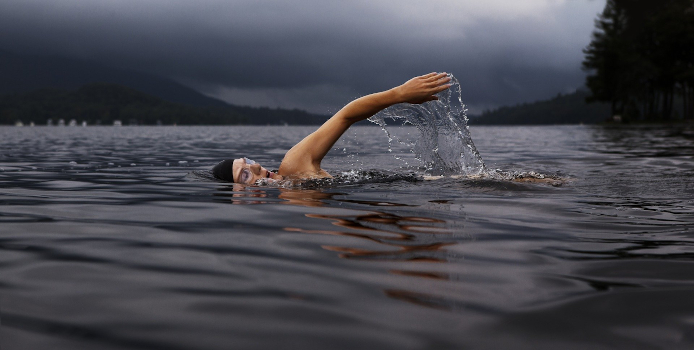Newton’s third law comes into play as a person swimming in the river experiences equal and opposite forces | Image: Stock photo, unknown source[●]

Newton’s third law can be observed when swimmers are in a river. As swimmers push the water backward with their hands, exerting an action force, they experience an equal and opposite reaction force from the water, pushing them forward. This exemplifies Newton’s third law, which states that every action force has an equal and opposite reaction force.

### BirdThe principle of Newton’s third law applies when a bird flying in the sky encounters equal and opposite forces | Image: Stock photo, unknown source[●]

When observing a flying bird, Newton’s third law of motion becomes evident. The bird’s wings generate an action force by pushing the air downwards, which results in an equal and opposite reaction force exerted by the air on the wings in an upward direction. This reciprocal interaction showcases the fundamental principle of Newton’s third law: for every action force, there is an equal and opposite reaction force.

### Jumping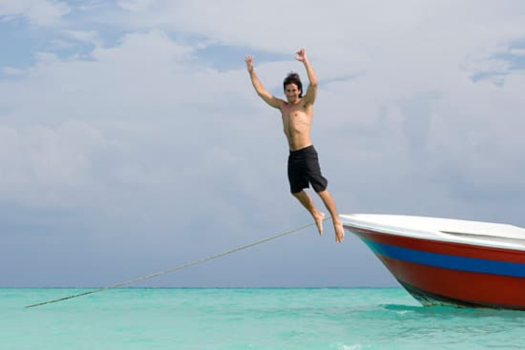Newton’s third law is demonstrated when a boy jumps off the boat and experiences equal and opposite forces | Image: Stock photo, unknown source[●]

Newton’s third law of motion is evident when observing an individual jumping off a boat. As the individual propels themselves off the boat, their leg exerts a force that pushes the boat backward, and in response, the boat exerts an equal and opposite reaction force on the individual’s leg, propelling them forward. This reciprocal interaction showcases how Newton’s third law dictates that every action force has an equal and opposite reaction force.

### Walking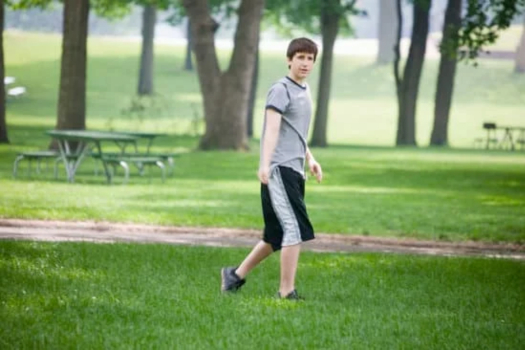When a boy walks on the ground, the application of Newton’s third law results in equal and opposite forces | Image: Stock photo, unknown source[●]

Newton’s third law of motion is observed when an individual walks on the ground. During walking, the legs exert a force that pushes the ground backward, representing the action force. In response, the ground exerts an equal and opposite reaction force on the legs. This interaction exemplifies Newton’s third law, which states that for every action force, there exists an equal and opposite reaction force.

### Rocket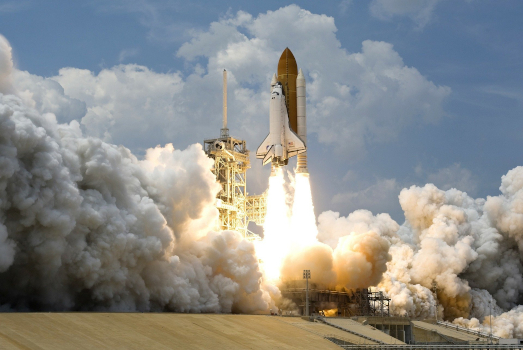The takeoff of a rocket from the ground involves the application of Newton’s third law, with equal and opposite forces in action | Image: Stock photo, unknown source[●]

Newton’s third law, which states that every action force has an equal and opposite reaction force, is evident during a rocket launch. When a rocket takes off from the ground, the action force is exerted by the rocket engines expelling exhaust gases, propelling the rocket forward. Simultaneously, an equal and opposite reaction force is exerted by the expulsion of these gases in the opposite direction, allowing the rocket to overcome the force of gravity and ascend into space. This interaction between the rocket and the expelled gases exemplifies Newton’s third law, demonstrating the equal and opposite nature of forces in action.

### Cannon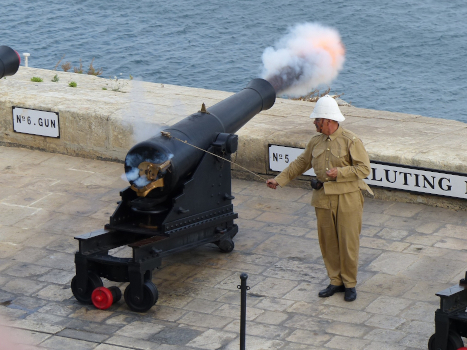A recoiling cannon showcases Newton’s third law in action, with equal and opposite forces exerted | Image: Stock photo, unknown source[●]

Newton’s third law can be seen in action when a cannon fires a cannonball. According to the third law of motion, every action force has an equal and opposite reaction force. In this case, the action force is exerted by the expanding gases in the cannon chamber, propelling the cannonball forward. Simultaneously, the cannonball exerts an equal and opposite reaction force on the gases, pushing them backward. This interaction between the cannon and the cannonball demonstrates the application of Newton’s third law, where forces always occur in pairs with equal magnitudes and opposite directions.

### Fish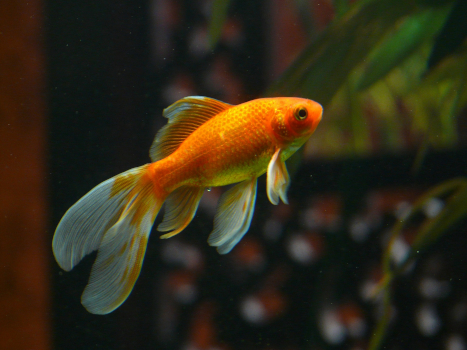Newton’s third law is demonstrated as a fish swims in the water, experiencing equal and opposite forces | Image: Stock photo, unknown source[●]

Newton’s third law is understood when observing fish swimming in water, as it states that every action force has an equal and opposite reaction force. In the case of fish swimming, the fish exerts a force on the water by flapping its fins or wings backward, creating an action force. Simultaneously, the water exerts an equal and opposite reaction force on the fish’s fins or wings, propelling the fish forward. This interaction between the fish and the water exemplifies the fundamental principle of Newton’s third law, where forces act in pairs with equal magnitudes and opposite directions.

### Spring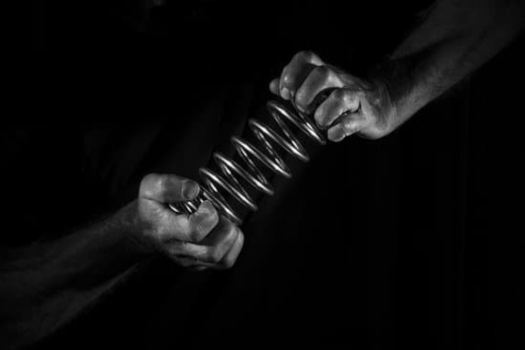When a hand presses a spring, Newton’s third law states that equal and opposite forces are exerted | Image: Stock photo, unknown source[●]

Newton’s third law can be seen in action when a spring is pressed with a hand. This fundamental law states that every action force has an equal and opposite reaction force. When a hand exerts a force to compress the spring inward, an action force is applied. Simultaneously, the spring exerts an equal and opposite reaction force, pushing outward. This interaction between the hand and the spring exemplifies Newton’s third law, where forces always occur in pairs with equal magnitudes and opposite directions.

### Boxing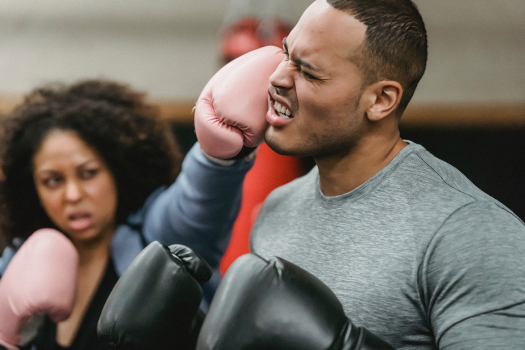In the sport of boxing, the opponent’s face experiences equal and opposite forces according to Newton’s third law | Image: Stock photo, unknown source[●]

In boxing, Newton’s third law is evident as every action force generates an equal and opposite reaction force. When a boxer delivers a punch to their opponent’s face, the force exerted by the boxer’s fist acts as the action force, resulting in an equal and opposite reaction force exerted by the opponent’s face on the boxer’s fist. This interaction showcases the fundamental principle of Newton’s third law, illustrating how forces occur in pairs with equal magnitudes but opposite directions.

### BoatRowing a boat with an oar showcases the application of Newton’s third law, resulting in equal and opposite forces | Image: Stock photo, unknown source[●]

While rowing a boat, Newton’s third law comes into play, stating that every action force has an equal and opposite reaction force. In this case, as the oar pushes against the water, it exerts an action force, propelling the boat forward. Simultaneously, the water exerts an equal and opposite reaction force on the oar, pushing it in the opposite direction. This interaction between the boat and the water exemplifies the fundamental principle of Newton’s third law, where forces are always paired, equal in magnitude, and opposite in direction.

### Ball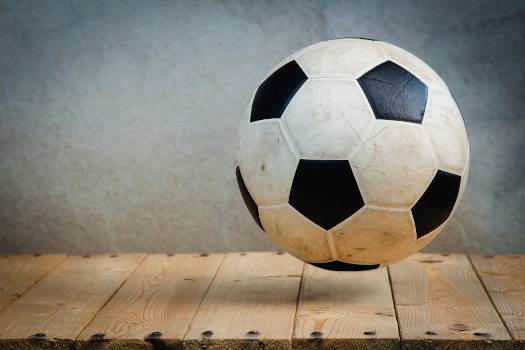A ball bouncing on the floor demonstrates Newton’s third law, with equal and opposite forces in action | Image: Stock photo, unknown source[●]

Newton’s third law is demonstrated when a ball bounces on the floor. During the bounce, the ball exerts a downward action force on the floor, and in response, the floor exerts an equal and opposite reaction force on the ball, propelling it upward. This interaction showcases the essence of Newton’s third law, which states that for every action force, there is an equal and opposite reaction force.

### Gun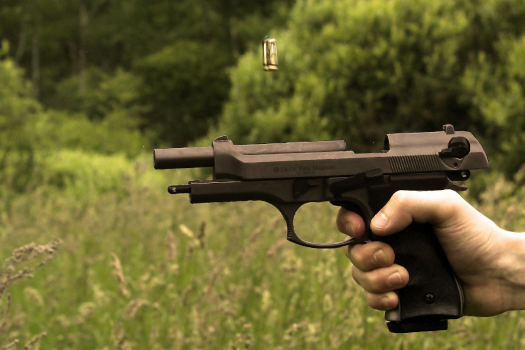The recoil of a gun serves as an example of Newton’s third law, with equal and opposite forces being exerted | Image: Stock photo, unknown source[●]

Newton’s third law is demonstrated when a gun fires a bullet. During the firing process, the expanding gases from the ignited gunpowder exert a forward action force on the bullet, propelling it out of the barrel. Simultaneously, the bullet exerts an equal and opposite reaction force on the gun in accordance with Newton’s third law. This interaction of forces exemplifies the fundamental concept that every action force has an equal and opposite reaction force.

### Pushing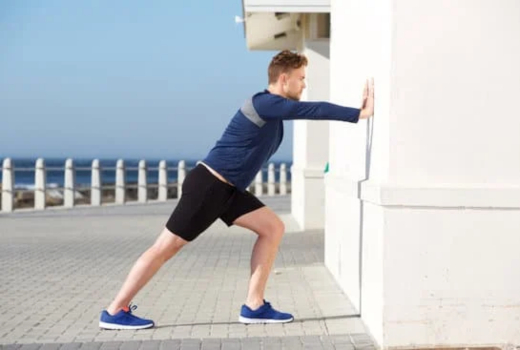When pushing a wall with the hand, Newton’s third law is demonstrated, as equal and opposite forces are at play | Image: Stock photo, unknown source[●]

Newton’s third law is demonstrated when pushing against a wall. When a force is exerted on the wall, such as by pushing with the hands, it acts as an action force, pushing the wall inward. Simultaneously, the wall exerts an equal and opposite reaction force, pushing back against the applied force. This interaction between the action force and the reaction force from the wall exemplifies the fundamental principle of Newton’s third law, where every action force has an equal and opposite reaction force.

## Image credit

• The stock photos used in this post are sourced from platforms like Pexels, Pixabay, Canva, etc. Due to the age of the images, their specific origins remain unknown.